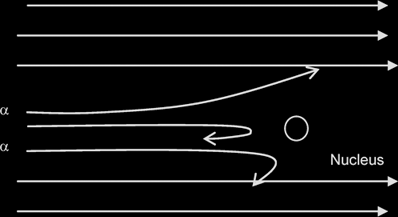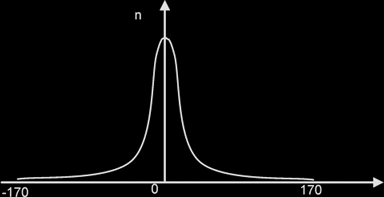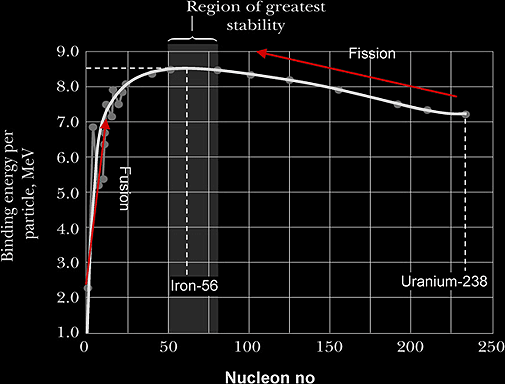# Nuclear Physics

Experimental evidence for a small charged nucleus in an atom:

Results of an experiment where a beam of alpha particles is fired at a thin gold foil, where n= no of alpha particles incident per unit time.Most of the α-particles passed through the metal foil were deflected by very small angles.

A very small proportion was deflected by more than 90°, some of these approaching 180°

From these 2 observations it can be deduced that: the nucleus occupies only a small proportion of the available space (ie the atom is mostly empty space) & that it is positively charged since the positively-charged alpha particles are repelled/deflected.

Nucleon: A particle within the nucleus; can be either a proton or a neutron

Nuclide: An atom with a particular number of protons and a particular number of neutrons

Proton number Z {old name: atomic number}: No. of protons in an atom

Nucleon number N {mass number}: Sum of number of protons and neutrons in an atom

Isotopes: are atoms with the same proton number, but different nucleon number {or different no of neutrons}

## Mass defect and Nuclear Binding Energy::

Energy & Mass are Equivalent: E = mc2 → ΔE = (Δm)c2

Nuclear Binding Energy:

• Energy that must be supplied to completely separate the nucleus into its individual nucleons/particles.

OR

• The energy released {not lost} when a nucleus is formed from its constituent nucleons.

B.E. per nucleon is a measure of the stability of the nucleus.

Mass Defect: The difference in mass between a nucleus and the total mass of its individual nucleons

Zmp + (A - Z)mn - Mass of Nucleus

Thus, Binding Energy. = Mass Defect x c2

In both nuclear fusion and fission, products have higher B.E. per nucleon {due to shape of BE per nucleon-nucleon graph}, energy is released {not lost} and hence products are more stable.

Energy released = Total B.E. after reaction (products) - Total B.E. before reaction (reactants)

Nuclear fission: The disintegration of a heavy nucleus into 2 lighter nuclei. Typically, the fission fragments have approximately the same mass and neutrons are emitted.

Fig below shows the variation of BE per nucleon plotted against the nucleon no.Warning!!! Graph is NOT symmetrical.

Principle of Conservation of Energy-Mass:

Total energy-mass before reaction = Total energy - mass after reaction
Σ (mc2 + ½ mv2)reactants = Σ (mc2 + ½ mv2)products + hf {if γ - photon emitted}

Energy released in nuclear reaction= Δmc2 = (Total rest mass before reaction – Total rest mass after reaction) x c2

Radioactivity is the spontaneous and random decay of an unstable nucleus, with the emission of an alpha or beta particle, and is usually accompanied by the emission of a gamma ray photon.

Spontaneous: The emission is not affected by factors outside the nucleus

Random: It cannot be predicted when the next emission will occur {Evidence in fluctuation in count-rate}

Decay law: dN/dt = -λN, where N= No. of undecayed {active} nuclei at that instant;

N = N0e-λt ; A = A0e-λt ; C = C0e-λt

Background radiation refers to radiation from sources other than the source of interest.

True count rate = Measured count rate – Background count rate

Nature of α, β & γ:

Alpha Particles Beta particles Gamma Particles
Notation α β γ
Charge + 2e - e No charge
Mass 4u 1/1840 u Massless
Nature Particle {He nucleus} Particle {electron emitted from nucleus} Electromagnetic Radiation
Speed Monoenergetic
(i.e. one speed only)
Continuous range
(up to approximately 98% of light)
100% speed of light

Decay constant λ is defined as the probability of decay of a nucleus per unit time {or,the fraction of the total no. of undecayed nuclei which will decay per unit time}

Activity is defined as the rate at which the nuclei are disintegrating; A = dN/dt = λN

A0 = λ N0

Number of undecayed nuclei ∝ Mass of sample

Number of nuclei in sample = (Sample Mass / Mass of 1 mol) x NA

where, Mass of 1 mol of nuclide= Nucleon No {or relative atomic mass} expressed in grams {NOT: in kg!!}

{Thus for eg, mass of 1 mole of U-235 = 235 g = 235 x 10-3kg, NOT: 235 kg}

Application of PCM to radioactive decay:

It is useful to remember that when a stationary nucleus emits a single particle, by PCM, after the decay,the
ratio of their KE = ratio of their speeds = reciprocal of the ratio of their masses

Half-life is defined as the average time taken for half the number {not: mass or amount} of undecayed nuclei in the sample to disintegrate,

or, the average time taken for the activity to be halved.

t½ = (ln2) / λ

Example:
Antimony-124 has a half-life of 60 days. If a sample of antimony-124 has an initial activity of 6.5 x 106Bq, what will its activity be after 1 year (365 days)?

Using A = A0e-λt & t½ = (ln2) / λ
A = 9.6 x 104Bq